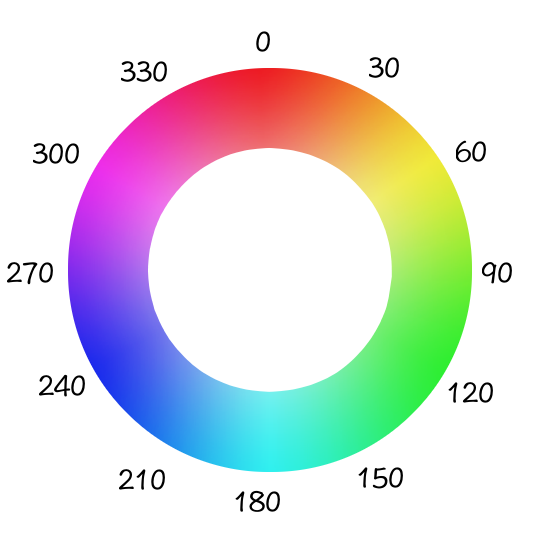# CSS hue-rotate() Function

Use the `hue-rotate()` function to apply a hue rotation on an image.

The CSS `hue-rotate()` function is used with the `filter` property to apply a hue rotation an image.

A hue rotation is where you specify an angle around the color circle that the input samples will be adjusted by.

The CSS `hue-rotate()` function requires an argument to tell it how much to rotate the hue by.

## Basic Example

Here's an example of a hue rotation:

## Negative Values

You can also use negative values to rotate in a counter-clockwise direction:

## How does Hue Rotation Work?

HSL (which stands for Hue Saturation Lightness) is a hue-based representation of the RGB color space of computer graphics. The HSL model is widely considered to be more intuitive than the RGB model. This is because, the HSL model allows you to select a base hue, and then adjust its saturation and lightness as desired.

Choosing a hue is a simple matter of selecting a spot on the color wheel. For example, red is at 0 degrees, blue is at 240 degrees, etc.When you use `hue-rotate()` to rotate the hue, you're rotating around this color circle. So you can see that red is at 0 degrees on the color circle. If your image contains red, and you rotate `240deg`, that part of the image will become blue.

## Official Syntax

The official syntax of the `hue-rotate()` function is as follows:

## Possible Values

The `hue-rotate()` function requires an angle value to specify how far the hue should be rotated.

You can use any of the following unit identifiers when specifying the angle:

`deg`
Degrees. There are 360 degrees in a full circle.
`grad`
Gradians, also known as "gons" or "grades" (although these aren't valid unit identifiers — you should still use `grad` as the unit identifier). There are 400 gradians in a full circle.
`rad`
`turn`
The unit identifier is optional for zero angles. For example, both `rotate(0deg)` and `rotate(0)` are valid (but `rotate(45)` is not).